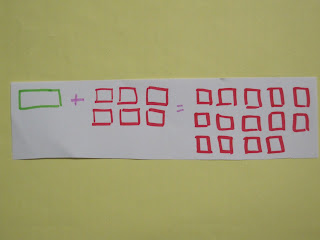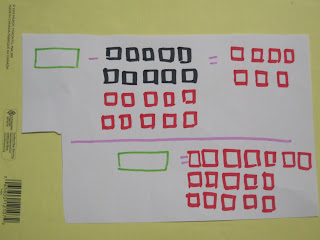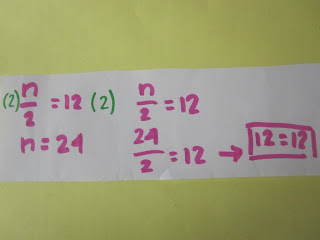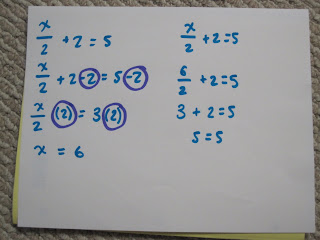## Sunday, May 8, 2011

### Melanie's Algebra Post

Some terms worth remembering:
• variable: is a letter that represents an unknown number in algebra (ex: a, q, t, k)
• constant: is the integer in an expression or equation (ex: x +6)
• expression: is a pattern and has many different answers (ex: 2x)
• equation: has an equals sign and has only one possible answer (ex: 2x=6)

How to solve One Step Algebra Equations:

1) Isolate the variable
2) Cancel using the opposite (cancel the constant using a zero pair)
3) Balance both sides (do the same on both sides of the equal sign)
4) Verify to solve equality

** Here are some examples of different types of One Step Algebra Equations:Subtraction (with Alge-tiles):Multiplication:Division:How to solve Two Step Algebra Equations:

1) Isolate the variable
2) Cancel the constant
3) Balance both sides of the equals sign
4) Simplify the variable
5) Balance both sides of the equals sign again
6) Verify to check

** Here are some examples of Two Step Algebra Equations:

Multiplication:Division: